• 有向图的强连通分量
万次阅读 多人点赞
2019-07-26 18:01:13

1 问题描述
引用自百度百科：

如果两个顶点可以相互通达，则称两个顶点强连通(strongly connected)。如果有向图G的每两个顶点都强连通，称G是一个强连通图。有向图的极大强连通子图，称为强连通分量(strongly connected components)。

Tarjan算法是基于对图深度优先搜索的算法，每个强连通分量为搜索树中的一棵子树。搜索时，把当前搜索树中未处理的节点加入一个堆栈，回溯时可以判断栈顶到栈中的节点是否为一个强连通分量。
定义DFN(u)为节点u搜索的次序编号(时间戳)，Low(u)为u或u的子树能够追溯到的最早的栈中节点的次序号。
当DFN(u)=Low(u)时，以u为根的搜索子树上所有节点是一个强连通分量。

2 解决方案
下面代码所使用图：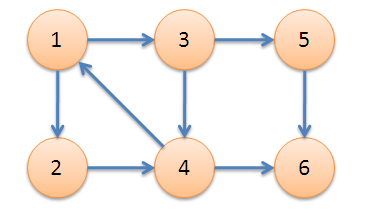package com.liuzhen.practice;

import java.util.ArrayList;
import java.util.Scanner;
import java.util.Stack;

public class Main {
public static int MAX = 100;
public static int count;   //用于对图中顶点遍历的次序进行计数
public static int n;
public static int[] DFN = new int[MAX];    //记录图中每个节点的DFS遍历的时间戳(即次序)
public static int[] Low = new int[MAX];   //记录每个顶点的所在树的根节点编号
public static boolean[] inStack = new boolean[MAX];  //用于记录当前节点是否在栈中
public static Stack<Integer> stack;

public void init(int n) {
count = 0;
stack = new Stack<Integer>();
for(int i = 0;i <= n;i++) {
DFN[i] = -1;   //代表顶点i未被遍历
Low[i] = -1;
inStack[i] = false;
}
}

static class edge {
public int a;  //边的起点
public int b;  //边的终点

edge(int a, int b) {
this.a = a;
this.b = b;
}
}

public void dfs(ArrayList<edge>[] map, int start) {
DFN[start] = count++;
Low[start] = DFN[start];
stack.push(start);
inStack[start] = true;
int j = start;
for(int i = 0;i < map[start].size();i++) {
j = map[start].get(i).b;
if(DFN[j] == -1) {  //顶点j未被遍历
dfs(map, j);
Low[start] = Math.min(Low[start], Low[j]);
} else if(inStack[j]) {
Low[start] = Math.min(Low[start], DFN[j]);
}
}
if(DFN[start] == Low[start]) {
System.out.print("强连通分量：");
do {
j = stack.pop();
System.out.print(j+" ");
inStack[j] = false;
} while(start != j);
System.out.println();
}
return;
}

public static void main(String[] args) {
Main test = new Main();
Scanner in = new Scanner(System.in);
n = in.nextInt();
test.init(n);
int k = in.nextInt();  //有向图的边数目
@SuppressWarnings("unchecked")
ArrayList<edge>[] map = new ArrayList[n + 1];
for(int i = 0;i <= n;i++)
map[i] = new ArrayList<edge>();
in.nextLine();
for(int i = 0;i < k;i++) {
int a = in.nextInt();
int b = in.nextInt();
}
test.dfs(map, 1);
}
}


运行结果：

8
2
3
4
4
5
1
6
6
强连通分量：6
强连通分量：5
强连通分量：3 4 2 1Java
更多相关内容
• 课 程 设 计 报 告 课程设计名称数据结构课程设计 课程设计题目实现求有向图强连通分量的算法 院系 专 班 学 姓 业 级 号 名 指导教师 沈阳航空航天大学课程设计报告 目 录 系 统 分 析 .1 1.1 题 目 介 绍 .1 1.2 ...
• 在键盘上输入有向图，对任意给定的图（顶点数和边数自定），建立它的邻接表并输出。然后判断该图是否连通。如果是连通图，求出该图的所有强连通分量并输出字符
• 文章目录有向图强连通分量1.定义：2.基本术语与概念2.1 边的概念2.2 缩点2.3 时间戳3. tarjan求强连通分量（SCC）3.1 原理3.2 步骤3.3 模板3.3.1 tarjan求强连通分量的过程3.3.2 缩点的过程4.例题题目1：P2341 ...

# 有向图强连通分量

## 1.定义：

给定一张有向图，若对于图中任意两个节点x，y，既存在从x到y的路径，也存在y到x的路径，则该有向图是强连通图。
有向图的极大强连通子图被称为：强连通分量，简称SCC(Strongly connected component)
Tarjan 算法基于dfs，可以在线性时间内求出一张有向图的所有连通分量。

## 2.基本术语与概念

### 2.1 边的概念

1.树枝边：搜索树中给定两个点x,y，x是y的父亲节点，那么这条边就是树枝边

2.前向边：搜索树中给定两个点x,y，x是y的祖先节点，那么这条边就是前向边

3.后向边：搜索树中给定两个点x,y，y是x的祖先节点，那么这条边就是后向边

4.横叉边：搜索树中给定两个点x,y，不属于以上三种的边，就叫横叉边，且y在搜索树中的编号一定小于x在搜索树中的编号。即dfn[y]<dfn[x]，（连向其他搜索过的节点的边），可以发现：往左边的横叉边是横叉边，往右边的横叉边实际上是树枝边，因为右边的节点还没有被搜过。

举个例子：

dfn数组存储的即是各个深度优先遍历过程中，每个节点第一次被访问的时间顺序，读者不妨对该图做一次深度优先遍历加以验证。
例如：1,2即是树枝边，注意：树枝边一定也是前向边
1,8即是前向边，4,6即是后向边，8,7是横叉边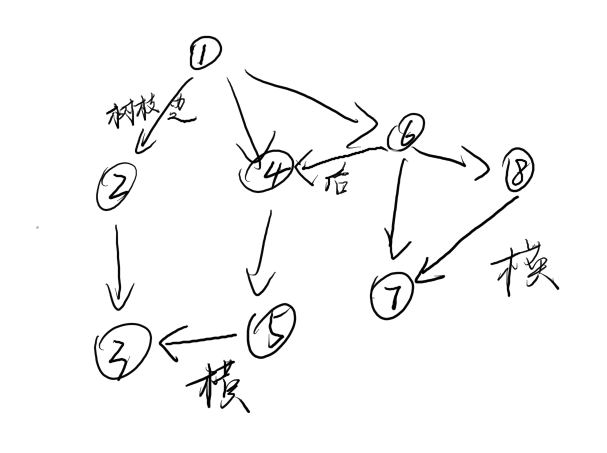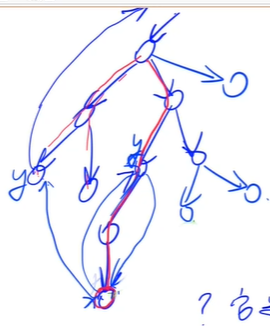### 2.2 缩点

将所有连通分量缩成一个点。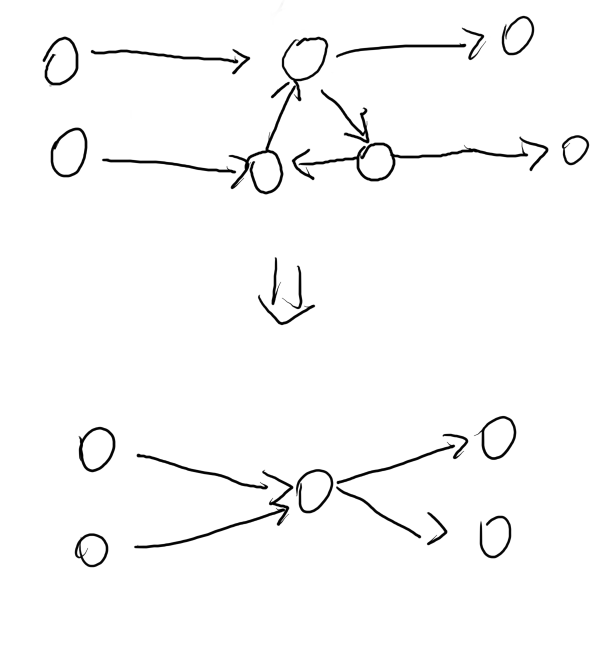### 2.3 时间戳

在搜索的时候，按照深度优先遍历顺序给每个点一个编号，即时间戳。
dfn[u]表示遍历到u的时间戳。

## 3. tarjan求强连通分量（SCC）

### 3.1 原理

1. 把边分成四大类：树枝边，前向边，后向边，横叉边
2. 取一个点x，判断它是否在强连通分量中
情况1：存在一条后向边使得它可以走到某个祖先节点
情况2：存在一条横叉边，它先通过横叉边，然后横叉边所在的点可以走到某个祖先节点
以上两种情况，点x和它的祖先节点之间所有节点都可以互相到达，满足强连通分量的定义。

### 3.2 步骤

1. 给每个点定义两个时间戳：
dfn[u]表示遍历到u的时间戳，即dfs的搜索顺序
low[u]表示从u开始走，所能遍历到的最小时间戳
2. u是其所在强连通分量的最高点，等价于dfn[u]==low[u]

### 3.3 模板

#### 3.3.1 tarjan求强连通分量的过程

void tarjan(int u)
{
dfn[u] = low[u] = ++timestamp;//时间戳
stk[++top] = u,in_stk[u] = true;//把当前点放到栈里面去，随后标记u已经入栈
for(int i = h[u];~i;i = ne[i])
{
int j = e[i];
if(!dfn[j])
{
tarjan(j);//先看一下u能到的点
//这里做完tarjan(j)以后，low[j]已经被更新了，由定义可知，low[j]保存的就是从j出发能到达的时间戳最小的点
low[u] = min(low[u],low[j]);//更新low[u]
}
else if(in_stk[j])
{
//可能通过其他边能搜到j，但不是当前分支搜到的，读者这里可以好好考虑考虑
//stk不一定只存了当前分支的点，还可能存有前面其他分支的点，比如横叉边所到达的点
//stk存的是当前还没有遍历完的强连通分量的所有点
low[u] = min(low[u],dfn[j]);
}
}
if(dfn[u]==low[u])
{
//u必然是u所在的强连通分量的最高点（由定义）
int y;
++scc_cnt;
do{
y = stk[top--];//把栈顶弹出
in_stk[y] = false;//标记它没有在栈里面
id[y] = scc_cnt;//标记y这个点属于scc_cnt这个强连通分量
}while(y!=u);
}
}
// y总原话：《最好背过》 :)


#### 3.3.2 缩点的过程

for(int i = 1;i<=n;i++)
{
for(i的所有邻点j)
{
if(i和j不在同一个强连通分量中)
{
把i所在的强连通分量向j所在的强连通分量加一条边;
}
}
}
//缩完点就是DAG(有向无环图)


注：缩完点以后，连通分量编号递减的顺序一定是拓扑序

给出注的证明:
对于一个图做一遍深度优先遍历，把每个点加入序列seq中
显然有seq的逆序即是拓扑序。

for(int i = h[u];~i;i = ne[i])
{
int j = e[i];
if(!st[j])
{
seq<-j;//把j存入seq序列中
dfs(j);
}
}
//读者不妨验证一下


最后，观察tarjan求有向图强连通分量的过程实际上就是对图做深度优先遍历的过程,所以最后按连通分量编号递减的顺序输出一定是拓扑序

## 4.例题

### 题目1：P2341 [USACO03FALL][HAOI2006]受欢迎的牛 G

一个思路是建反图，对每个奶牛做一遍dfs，统计完美奶牛个数，时间复杂度O(nm)，显然超时。
再观察：
题意知当是有向无环图时，只要有两个点出度为0，那么完美奶牛个数为0，如果有一个出度为0，那么完美奶牛个数为1，
于是可以tarjan缩点，如果只有一个出度为0的强连通分量，那么答案就是该强连通分量的size。

#include<bits/stdc++.h>
using namespace std;
const int N = 1e4+10,M = 5e4+10;
int n,m;
int h[N], e[M], ne[M], idx;
int dfn[N], low[N], timestamp;  // 时间戳
int stk[N], top;
bool in_stk[N];
int id[N], scc_cnt;  // 每个点所属分量编号
int dout[N],sz[N];//出度数组

{
e[idx] = b,ne[idx] = h[a],h[a] = idx++;
}

void tarjan(int u)
{//缩点
dfn[u] = low[u] = ++timestamp;
stk[++top] = u,in_stk[u] = true;
for(int i = h[u];~i;i = ne[i])
{
int j = e[i];
if(!dfn[j])
{
tarjan(j);
low[u] = min(low[u],low[j]);
}
else if(in_stk[j])
{
low[u] = min(low[u],dfn[j]);
}
}
if(dfn[u]==low[u])
{
++scc_cnt;
int y;
do{
y = stk[top--];
in_stk[y] = false;
id[y] = scc_cnt;
sz[scc_cnt]++;
}while(y!=u);
}
}
int main()
{
memset(h, -1, sizeof h);
cin>>n>>m;
while (m -- ){
int a,b;
cin>>a>>b;
}
for(int i = 1;i<=n;i++)
{
if(!dfn[i])
{
tarjan(i);
}
}
for(int i = 1;i<=n;i++)
{
for(int j = h[i];~j;j = ne[j])
{
int k = e[j];
int a = id[i],b = id[k];
if(a!=b)
{
//说明i和j不在同一个连通分量中
dout[a]++;//这里没有实际把边加出来，因为不在同一个连通分量中，所以要把a的出度+1，等价于加了一条边
}
}
}
int sum = 0,zeros = 0;//zeros记录出度为0的连通分量的个数
for(int i = 1;i<=scc_cnt;i++)
{
if(!dout[i])
{
zeros++;
sum+=sz[i];
if(zeros>1)
{
cout<<0;
return 0;
}
}
}
cout<<sum;
return 0;
}


### 题目2：P2746 [USACO5.3]校园网Network of Schools

第一个问类似题目1，第二个问需要找小性质：如何让有向无环图变成强连通图添加的边的数量最少？
设 a 为 入 度 为 0 的 点 的 个 数 ， b 为 出 度 为 0 的 点 的 个 数 那 么 易 有 a n s = m a x ( a , b ) 设a为入度为0的点的个数，b为出度为0的点的个数\\ 那么易有ans = max(a,b)
题解来自《算法竞赛进阶指南》：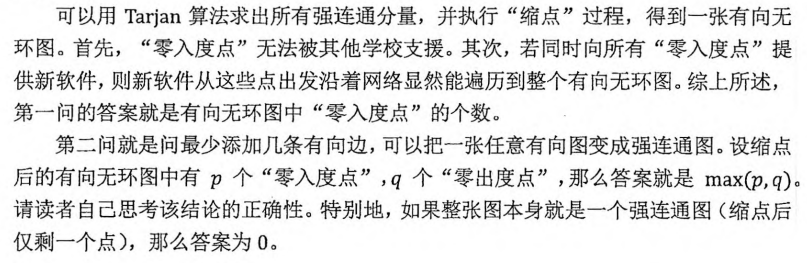AC code:

#include<bits/stdc++.h>
using namespace std;
const int N = 1e4+10,M = 5e4+10;
int n,m;
int h[N], e[M], ne[M], idx;
int dfn[N], low[N], timestamp;  // 时间戳
int stk[N], top;
bool in_stk[N];
int id[N], scc_cnt;  // 每个点所属分量编号
int dout[N],sz[N],din[N];//出度数组

{
e[idx] = b,ne[idx] = h[a],h[a] = idx++;
}

void tarjan(int u)
{//缩点
dfn[u] = low[u] = ++timestamp;
stk[++top] = u,in_stk[u] = true;
for(int i = h[u];~i;i = ne[i])
{
int j = e[i];
if(!dfn[j])
{
tarjan(j);
low[u] = min(low[u],low[j]);
}
else if(in_stk[j])
{
low[u] = min(low[u],dfn[j]);
}
}
if(dfn[u]==low[u])
{
++scc_cnt;
int y;
do{
y = stk[top--];
in_stk[y] = false;
id[y] = scc_cnt;
sz[scc_cnt]++;
}while(y!=u);
}
}
int main()
{
memset(h, -1, sizeof h);
cin>>n;
for(int i = 1;i<=n;i++)
{
int x;
while(cin>>x,x)
{
}
}
for(int i = 1;i<=n;i++)
{
if(!dfn[i])
{
tarjan(i);
}
}
for(int i = 1;i<=n;i++)
{
for(int j = h[i];~j;j = ne[j])
{
int k = e[j];
int a = id[i],b = id[k];
if(a!=b)
{
//说明i和j不在同一个连通分量中
dout[a]++;//这里没有实际把边加出来，因为不在同一个连通分量中，所以要把a的出度+1，等价于加了一条边
din[b]++;
}
}
}
int sum1 = 0,sum2 = 0,cnt1 = 0,cnt2 = 0;
//cnt1记录出度为0的连通分量的个数
//cnt2记录入度为0的连通分量的个数
for(int i = 1;i<=scc_cnt;i++)
{
if(!dout[i])
{
cnt1++;
}
if(!din[i])
{
cnt2++;
}
}
if(scc_cnt!=1)
cout<<cnt2<<endl<<max(cnt1,cnt2);
else
cout<<cnt2<<endl<<"0";
return 0;
}



陆续更新~

## 5.参考资料

1. acwing算法提高课图论
2. 《算法竞赛进阶指南》
展开全文算法 深度优先
• 算法简介Tarjan 算法一种由Robert Tarjan提出的求解有向图强连通分量的算法，它能做到线性时间的复杂度。我们定义：如果两个顶点可以相互通达，则称两个顶点连通(strongly connected)。如果有向图G的每两个顶点都...

一.算法简介

Tarjan 算法一种由Robert Tarjan提出的求解有向图强连通分量的算法，它能做到线性时间的复杂度。

我们定义：

如果两个顶点可以相互通达，则称两个顶点强连通(strongly connected)。如果有向图G的每两个顶点都强连通，称G是一个强连通图。有向图的极大强连通子图，称为强连通分量(strongly connected components)。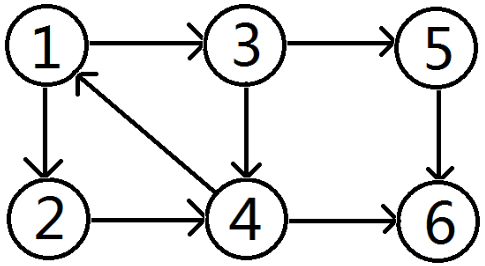例如：在上图中，{1 , 2 , 3 , 4 } , { 5 }　, { 6 } 三个区域可以相互连通，称为这个图的强连通分量。

Tarjan算法是基于对图深度优先搜索的算法，每个强连通分量为搜索树中的一棵子树。搜索时，把当前搜索树中未处理的节点加入一个堆栈，回溯时可以判断栈顶到栈中的节点是否为一个强连通分量。

再Tarjan算法中，有如下定义。

DFN[ i ] : 在DFS中该节点被搜索的次序(时间戳)

LOW[ i ] : 为i或i的子树能够追溯到的最早的栈中节点的次序号

当DFN[ i ]==LOW[ i ]时，为i或i的子树可以构成一个强连通分量。

二.算法图示

以1为Tarjan 算法的起始点，如图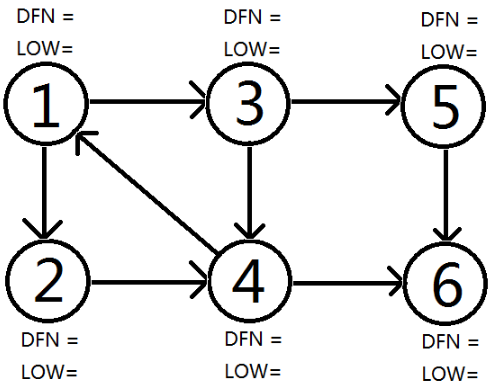顺次DFS搜到节点6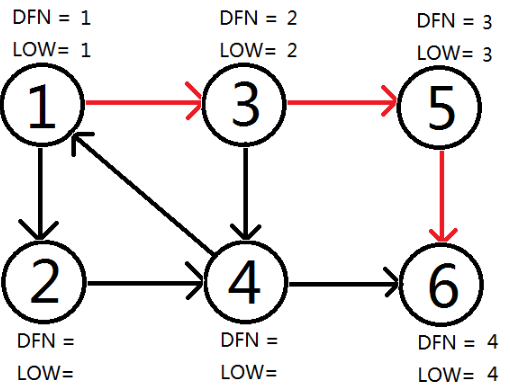回溯时发现LOW[ 5 ]==DFN[ 5 ] , LOW[ 6 ]==DFN[ 6 ] ,则{ 5 } , { 6 } 为两个强连通分量。回溯至3节点，拓展节点4.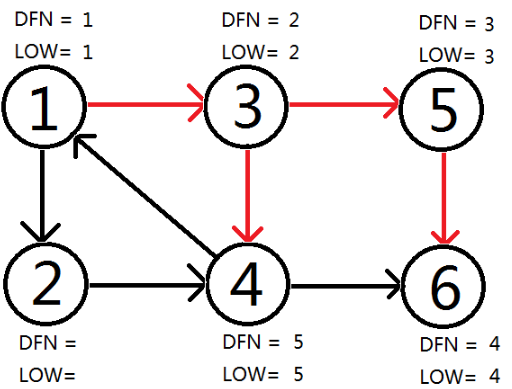拓展节点1 ， 发现1再栈中更新LOW[ 4 ]，LOW[ 3 ] 的值为1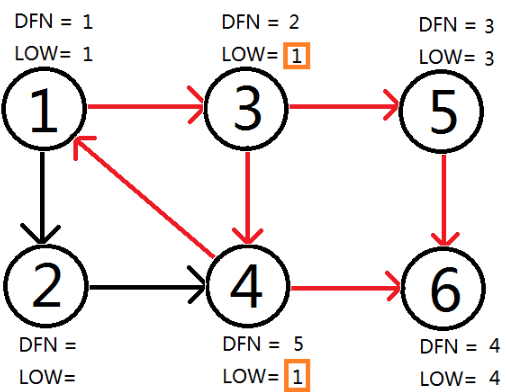回溯节点1，拓展节点2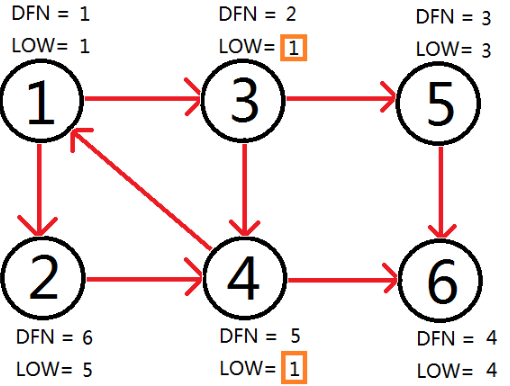自此，Tarjan Algorithm 结束，{1 , 2 , 3 , 4 } , { 5 }　, { 6 } 为图中的三个强连通分量。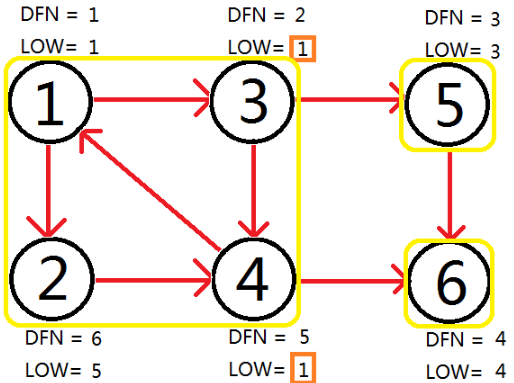不难发现，Tarjan Algorithm 的时间复杂度为O(E+V).

三.算法模板

先来一段伪代码压压惊：
tarjan(u){

DFN[u]=Low[u]=++Index // 为节点u设定次序编号和Low初值

Stack.push(u) // 将节点u压入栈中

for each (u, v) in E // 枚举每一条边

if (v is not visted) // 如果节点v未被访问过

tarjan(v) // 继续向下找

Low[u] = min(Low[u], Low[v])

else if (v in S) // 如果节点u还在栈内

Low[u] = min(Low[u], DFN[v])

if (DFN[u] == Low[u]) // 如果节点u是强连通分量的根

repeat v = S.pop // 将v退栈，为该强连通分量中一个顶点

print v

until (u== v)

}

复制代码

 void Tarjan ( int x ) {
dfn[ x ] = ++dfs_num ;
low[ x ] = dfs_num ;
vis [ x ] = true ;//是否在栈中
stack [ ++top ] = x ;
for ( int i=head[ x ] ; i!=0 ; i=e[i].next ){
int temp = e[ i ].to ;
if ( !dfn[ temp ] ){
Tarjan ( temp ) ;
low[ x ] = gmin ( low[ x ] , low[ temp ] ) ;
}
else if ( vis[ temp ])low[ x ] = gmin ( low[ x ] , dfn[ temp ] ) ;
}
if ( dfn[ x ]==low[ x ] ) {//构成强连通分量
vis[ x ] = false ;
color[ x ] = ++col_num ;//染色
while ( stack[ top ] != x ) {//清空
color [stack[ top ]] = col_num ;
vis [ stack[ top-- ] ] = false ;
}
top -- ;
}
}
  #include<cstdio>
#include<algorithm>
#include<string.h>
using namespace std;
struct node {
int v,next;
}edge;
int DFN,LOW;
{
edge[cnt].v = y;
return ;
}
void tarjan(int x)//代表第几个点在处理。递归的是点。
{
DFN[x]=LOW[x]=++tot;// 新进点的初始化。
stack[++index]=x;//进站
visit[x]=1;//表示在栈里
{
if(!DFN[edge[i].v]) {//如果没访问过
tarjan(edge[i].v);//往下进行延伸，开始递归
LOW[x]=min(LOW[x],LOW[edge[i].v]);//递归出来，比较谁是谁的儿子／父亲，就是树的对应关系，涉及到强连通分量子树最小根的事情。
}
else if(visit[edge[i].v ]){  //如果访问过，并且还在栈里。
LOW[x]=min(LOW[x],DFN[edge[i].v]);//比较谁是谁的儿子／父亲。就是链接对应关系
}
}
if(LOW[x]==DFN[x]) //发现是整个强连通分量子树里的最小根。
{
do{
printf("%d ",stack[index]);
visit[stack[index]]=0;
index--;
}while(x!=stack[index+1]);//出栈，并且输出。
printf("\n");
}
return ;
}
int main()
{
int n,m;
scanf("%d%d",&n,&m);
int x,y;
for(int i=1;i<=m;i++)
{
scanf("%d%d",&x,&y);
}
for(int i=1;i<=n;i++)
if(!DFN[i])  tarjan(1);//当这个点没有访问过，就从此点开始。防止图没走完
return 0;
}
展开全文算法
• 而一个有向图中的极大连通子图就被称为强连通分量。(极大连通子图就是指一个连通子图，再加入任何额外的节点都无法保证这个新的图是一个连通图) 举个例子： 显然这个图里两个点集[1,3,5][1,3, 5][1,3,5]和 ...

# 1.定义

在一个有向图中，如果有两个点 ( u , v ) (u,v) 可以在图中互相到达，那么就称这两个点是强联通的.。而如果一个有向图上的任意两点都可以互相到达，那么就成这个图为强连通图。而一个有向图中的极大强连通子图就被称为强连通分量。(极大强连通子图就是指一个强连通子图，再加入任何额外的节点都无法保证这个新的图是一个强连通图)

举个例子：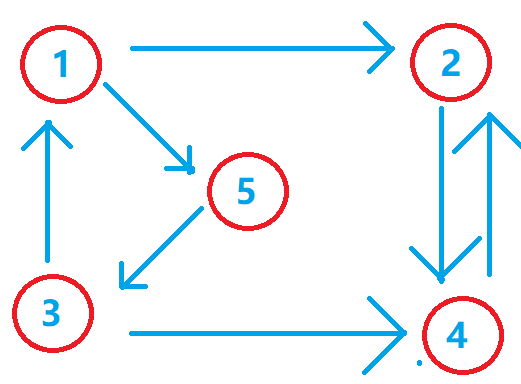显然这个图里两个点集 [ 1 , 3 , 5 ] [1,3, 5] [ 2 , 4 ] [2, 4] ，在各自点集里的各个点都可以互相到达，且不存在更大的点集，所以称这两个点集都是强连通分量。而 [ 1 , 2 ] [1, 2] 和[2, 3, 4] 都不可以任意点都互相到达，所以它们不是强连通分量。

# 2.用处

刚刚介绍了强连通分量的定义，现在来看看强连通分量有什么用吧。
一般强连通分量都是用来缩点的，什么是缩点？就是将一些可以互相到达的点缩成一个点，能够互相到达的点当然就是强连通分量啦。那么缩点的用处是什么呢？显然你会发现在一个有向图中，所有的强连通分量就是一个个的环。你把所有的强连通分量都缩成点之后，再建出来的图，就会建出一个拓扑图(ps. 拓扑图就是有向无环图) 出来。也就是说借助强连通分量你可以将任何有向图都建成一个拓扑图，这样就可以利用很多拓扑图的性质来解题了。比如求有向图的最长/短距离或者要递推某些变量的。
而且求强连通分量的板子基本是不变

# 3.Tarjan算法求强连通分量

目前Tarjan算法算是比较好理解且好写的求强连通分量的方法，复杂度和维护的性质都比较优秀，Tarjan算法的时间复杂度是线性的 O ( n + m ) O(n+m)

### (1)Tarjan的基本流程及简单证明

首先Tarjan解法是基于深度优先搜索进行遍历的算法，我们可以先假设一个dfs遍历节点的过程是类似一颗不会搜索重复节点的搜索树，那么强连通分量就是这棵树上的某几个子树。我们对这颗搜索树的边做几个定义。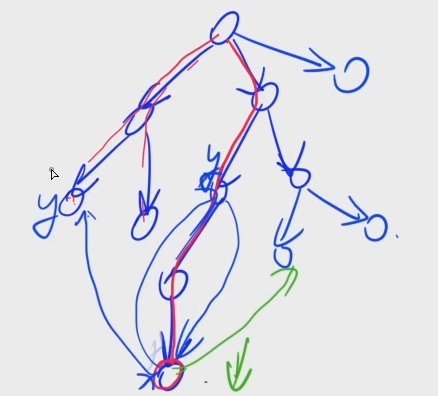1. 树枝边：即为按dfs顺序遍历到的所有的边即为树枝边
2. 前向边：这条边的一个点是另外一个点的祖先，且这条边是由深度低的点指向深度高的点(树枝边就是特殊的前向边)
3. 后向边：和前向边类似。但是是由深度高的点只想深度低的点。
4. 横插边：这条边上的两点是两颗子树上的点。且指向一定是由dfs后搜到的点指向先搜到的点

这些定义说实在的和我们讲的Tarjan并没有太大的关系…
Tarjan算法主要是引入了一个新的定义，即时间戳，时间戳说实在的就是dfs遍历的顺序，即dfs序。
Tarjan需要维护两个数组 d f n [ i ] dfn[i] l o w [ i ] low[i] 以及一个栈。 d f n [ i ] dfn[i] 即为搜索到的第i个节点的时间戳，而 l o w [ i ] low[i] 得先介绍完栈的用处之后再解释。栈是用来在dfs回溯中，判断某个节点是否在某个还未处理完的强连通分量的。也就是说栈中存储的是按dfs序的顺序存储的还没有处理完的强连通分量的点。一旦判断出某些点是强连通分量后，就会把这些点弹出。 l o w [ i ] low[i] 这个数组存储的是第 i i 个点包括 i i 点已及还在栈中的 i i 的子树能到达的时间戳最小的点的时间戳。

i i 点以及他的子树全部遍历完了之后，如果 l o w [ i ] low[i] d f n [ i ] dfn[i] 相等，那么也就是说， i i 这个点是包括这个点的强连通分量时间戳最低的点。那么他自然不可能再加入新的节点来使他的强连通分量变得更大，即包括第 i i 个结点的强连通分量已经搜完，就可以将这个结点从栈中退出来。

值得一提的是，现在栈顶到 i i 个结点之间的所有结点就是这个强连通分量里的结点，所以把栈顶到 i i 个结点之间的所有结点都退出来，他们构成一个强连通分量

证明： 因为首先 i i 结点是这个强连通分量里时间戳最小的一个，且这个过程是在回溯时进行的，故栈中堆顶的元素到 i i 点之间的结点必然是 i i 结点子树上的结点，并且在 i i 点之前的点肯定也不会是 i i 点连通分量里的点(想想 l o w low 的定义就明白了)。又根据 l o w [ i ] low[i] 的定义，可知这些结点的 l o w low 不可能小于 i i 点的 l o w low ，所以 i i 点子树中不属于 i i 点的连通分量的结点，它们所在的联通分量上的结点也只可能在 i i 点的子树内，而 i i 点的子树已经全部处理完了，所以，在回溯到 i i 点之前，那些不属于 i i 点联通分量的结点必然在之前已经处理完，并退出栈内。所以剩下的点必然都是属于 i i 点联通分量上的结点。（Tarjan牛逼啊）

### (2)代码模板

Tarjan算法的模板比较固定，基本上不会做太多的改变，所以一般做题的话，可以直接套板子处理有向图。

补充：一个点就是我看到很多人写完Tarjan后，还会再进行一次拓扑排序，其实完全没有必要，可以注意到，拓扑排序中有一种dfs的写法和Tarjan算法的做法很类似，所以Tarjan后缩点的图的拓扑序，就是这个缩点图的点编号的倒序输出。

vector<int> G[N], D[N];
int f[N], g[N];
int dfn[N], low[N], tim, idx, scc_idx; //tim为时间戳,idx为栈顶下标，scc_idx为强连通分量个数
int sta[N], sz[N], id[N];			//sta为栈，sz为缩点后的点里包括多少个点，id为缩点见图后的新下标
bool in_sta[N];						//in_sta为检查某个结点是否还在栈内

void tarjan(int u){
dfn[u] = low[u] = ++ tim;
sta[++idx] = u; in_sta[u] = true;
for(int i = 0;i < G[u].size();i++){
int v = G[u][i];
if(!dfn[v]){
tarjan(v);
low[u] = min(low[u],low[v]);
}
else if(in_sta[v]) low[u] = min(low[u],low[v]);
}
if(low[u] == dfn[u]){
scc_idx++;
int y;
do{
y = sta[idx--];
in_sta[y] = false;
id[y] = scc_idx;
sz[scc_idx]++;
}while(y != u);
}
}


### (3)例题

#### a. USACO 2003 Fall (受欢迎的牛)

##### 题目描述

每一头牛的愿望就是变成一头最受欢迎的牛。现在有 N 头牛，给你 M 对整数 (A,B)，表示牛 A 认为牛 B 受欢迎。这种关系是具有传递性的，如果 A 认为 B 受欢迎，B 认为 C 受欢迎，那么牛 A 也认为牛 C 受欢迎。你的任务是求出有多少头牛被除自己之外的所有牛认为是受欢迎的。

##### 输入

第一行两个数 N,M；

接下来 M 行，每行两个数 A,B，意思是 A 认为 B 是受欢迎的（给出的信息有可能重复，即有可能出现多个 A,B）。

##### 输出

输出被除自己之外的所有牛认为是受欢迎的牛的数量。

3 3
1 2
2 1
2 3

1

##### 数据范围：

对于全部数据， 1 ≤ N ≤ 1 0 4 , 1 ≤ M ≤ 5 × 1 0 4 1≤N≤10^4,1≤M≤5×10^4

##### 题解

对于这道题没啥好说的，缩完点直接出度为0的点有多少，超过1个的答案就是0。否则就是那个点的大小(大小就是缩点前的这个强连通分量包括几个点)

##### 代码
#include<bits/stdc++.h>
using namespace std;
const int N = 1e5+50;

vector<int> G[N];
int tim, sta[N], sz[N], id[N], idx;
int scc_idx, dfn[N], low[N], du[N];
bool in_sta[N];

void tarjan(int u){
dfn[u] = low[u] = ++tim;
sta[++idx] = u; in_sta[u] = true;
for(int i = 0;i < G[u].size();i++){
int v = G[u][i];
if(!dfn[v]){
tarjan(v);
low[u] = min(low[u], low[v]);
}
else if(in_sta[v]){
low[u] = min(low[u], low[v]);
}
}
if(low[u] == dfn[u]){
int y;
scc_idx++;
do{
y = sta[idx--];
in_sta[y] = false;
id[y] = scc_idx;
sz[scc_idx]++;
}while(u != y);
}
}

int main(){
int n, m;
scanf("%d %d",&n,&m);
while(m--){
int a, b;
scanf("%d %d",&a,&b);
G[a].push_back(b);
}
for(int i = 1;i <= n;i++){
if(!dfn[i]) tarjan(i);
}
for(int i = 1;i <= n;i++){
for(int j = 0;j < G[i].size();j++){
if(id[G[i][j]] != id[i]) du[id[i]]++;
}
}
int ans = 0, tmp = 0;
for(int i = 1;i <= scc_idx;i++){
if(du[i] == 0) ans = sz[i], tmp++;
}
if(tmp > 1) printf("0\n");
else printf("%d\n",ans);
return 0;
}


#### b.《算法竞赛进阶指南》, usaco training 5.3 （学校网络）

##### 题目描述

一些学校连接在一个计算机网络上，学校之间存在软件支援协议，每个学校都有它应支援的学校名单（学校 A 支援学校 B，并不表示学校 B 一定要支援学校 A）。

当某校获得一个新软件时，无论是直接获得还是通过网络获得，该校都应立即将这个软件通过网络传送给它应支援的学校。

因此，一个新软件若想让所有学校都能使用，只需将其提供给一些学校即可。

现在请问最少需要将一个新软件直接提供给多少个学校，才能使软件能够通过网络被传送到所有学校？

最少需要添加几条新的支援关系，使得将一个新软件提供给任何一个学校，其他所有学校就都可以通过网络获得该软件？

##### 输入格式

第 1 行包含整数 N，表示学校数量。

第 2…N+1 行，每行包含一个或多个整数，第 i+1 行表示学校 i 应该支援的学校名单，每行最后都有一个 0 表示名单结束（只有一个 0 即表示该学校没有需要支援的学校）。

##### 输出格式

输出两个问题的结果，每个结果占一行。

2≤N≤100

5
2 4 3 0
4 5 0
0
0
1 0

1
2

##### 题解

这个题目第一问很简单，就是缩点后入读为0的点的个数。
第二问的答案则是入读为0的点和出度为0的点取最大值。
这边只证明第二问：假设有 p p 和入读为0的点， q q 个初读为0的点，这边先假设 ( p < = q ) (p <= q) ( p > q ) (p > q) 的证明类似。
我们称出度为0的点为终点，入读为0的点为起点
首先可以肯定地是一个终点总有一个起点可以到达它。
我们先看 p = 1 p = 1 的时候

如果 p = 1 p = 1 那么也就是说这个起点可以到达所有的终点，即只要让每个终点都和起点连一个边即可即 q q 条边。

如果 p > 1 p > 1 那么必然可以找到两组不同的起点 ( q 1 , q 2 ) (q1,q2) 走到不同的终点 ( p 1 , p 2 ) (p1,p2) 。 即 ( q 1 − > p 1 ) (q1->p1) ( q 2 − > p 2 ) (q2->p2) 。于是我们总可以建一个 ( p 1 − > q 2 ) (p1->q2) 的边使得起点和终点各减少一个。最后会变为 q = 1 q = 1 的情况。所以发现我们总可以只建 m a x ( p , q ) max(p,q) 个边使得起成为整个图变成强连通分量。并且一定是最优的。

然后在特判一下，如果整个图只有一个强连通分量，那么第二问答案就是0。

##### 代码
//强联通分量可以把任意有向图转化为一个有向无环图(拓扑图)，并且使用tarjan 算法求出的强连通分量的编号逆向输出就是这个拓扑图的拓扑序
#include<bits/stdc++.h>
using namespace std;
const int N = 1e5+50;

vector<int> G[N];
bool in_sta[N];
int id[N], low[N], dfn[N], sta[N];
int tim, in[N], out[N], scc_idx, idx;

void tarjan(int u){
dfn[u] = low[u] = ++tim;
sta[++idx] = u; in_sta[u] = true;
for(int i = 0;i < G[u].size();i++){
int v = G[u][i];
if(!dfn[v]){
tarjan(v);
low[u] = min(low[u], low[v]);
}
else if(in_sta[v]) low[u] = min(low[u],dfn[v]);
}
if(low[u] == dfn[u]){
int y;
scc_idx++;
do{
y = sta[idx--];
in_sta[y] = false;
id[y] = scc_idx;
}while(y != u);
}
}

int main(){
int n;
scanf("%d",&n);
int ans1 = 0, ans2 = 0;
for(int i = 1;i <= n;i++){
int x;
while(scanf("%d",&x) && x != 0) G[i].push_back(x);
}
for(int i = 1;i <= n;i++){
if(!dfn[i]) tarjan(i);
}
for(int i = 1;i <= n;i++){
for(int j = 0;j < G[i].size();j++){
int v = id[G[i][j]];
if(v != id[i]) {
in[v]++; out[id[i]]++;
}
}
}
for(int i = 1;i <= scc_idx;i++){
if(in[i] == 0) ans1++;
if(out[i] == 0) ans2++;
}
printf("%d\n",ans1);
if(scc_idx == 1) printf("0\n");
else printf("%d\n",max(ans1,ans2));
return 0;
}
/*
5
2 4 3 0
4 5 0
0
0
1 0
*/

展开全文算法 深度学习 自动驾驶
• 有向图强连通分量 1.连通 代表的是 这个连通块中的每两个点互相都是有一条路径是可以走到的 2.分量 就是子图； 从这张图上可以看出 A B C这三个点都是互相可以走到的 所以他们就是一个联通块 D E F 三个点都是...c++
• [有向图强连通分量] 在有向图G中，如果两个顶点间至少存在一条路径，称两个顶点连通(strongly connected)。...非连通图有向图的极大连通子图，称为强连通分量(strongly connected components)。 下图中，子...
• 对于一个图，说他是连通的当且仅当从该图的任何一个顶点到该图的另一个顶点都走得通，连通的意思是，对于一个有向图，任何一个顶点到另外一个顶点都能互通，强连通分量就是极大连通子图，可以这么理解，对于该图...
• 浅谈无向图的连通连通图是无向图的一个概念： 在无向图中，若从顶点 v1v_1v1​ 到顶点 v2v_2v2​ 有路径，则称顶点 v1v_1v1​ 与 v2v_2v2​ 是连通的； 如果图中任意一对顶点都是...有向图强连通性的概念 在有向图算法 数据结构
• 有向图中，如果一些顶点中任意两个顶点都能互相到达（间接或直接）,那么这些顶点就构成了一个强连通分量，如果一个顶点没有出度，即它不能到达其他任何顶点，那么该顶点自己就是一个强连通分量。 做题的总结...
• 一、什么是有向图强连通分量？ 和无向图的点双连通分量相似（但这里可以一个点可以经过多次），在一个极大连通分量中，任意两个结点u,v ,有u 到 v的路径，也有v 到 u 的路径，也就是在有向边的条件下，在该极大...算法
• 强连通分量】非连通图有向图 的 极大连通子图 算法 使用【DFS】 步骤一 思路 有向图G上，从某个顶点出发沿以【该顶点】为【尾的弧】进行DFS 并按其【所有邻接点】的【搜索都...
• 正如标题所介绍的那样，Tarjan算法的目标就是找出图中的连通图的，其实前提条件是这样的，在一个有向图中，有时必然会出现节点成环的情况，而在图内部的这些形成环的节点所构成的图就是我们所要找算法
• [有向图强连通分量] 在有向图G中，如果两个顶点间至少存在一条路径，称两个顶点连通(strongly connected)。...非连通图有向图的极大连通子图，称为强连通分量(strongly connected components)。 下图
• 有向图连通分量的求解思路 kosaraju算法 逛了很多博客，感觉都很难懂，终于找到一篇能看懂的，摘要记录一下 原博客https://www.cnblogs.com/nullzx/p/6437926.html 关于连通分量是什么自行百度，这里主要...kosaraju算法
• 有向图强连通分量（SCC） 1. 有向图强连通分量原理 原理 强连通分量是针对有向图来说的。如下的讲解默认都是针对有向图的。 连通分量：对于一个有向图中的一些点来说，如果任意两点都能相互到达，则称这些...
• 对于一个有向图, 连通分量: 对于分量中任意u, v, 必然可以从u走到v, 也可以从v走到u (极大)连通分量 极大连通分量. 如果连通分量加上任何一个点之后, 都不是连通分量了, 那么称这个连通分量为极大连通分量(强连通...
• 如何找到有向图（a）中的所有强连通分量，如图（b) 二、kosaraju算法 Kosaraju的算法（又称为–Sharir Kosaraju算法）是一个线性时间(linear time)算法找到的有向图强连通分量。 1. 原理 它利用了一个...
• 考虑强连通分量C，设其中第一个被发现的点为x，则，C中其他的点都是x的后代。我们希望在x访问完成时立即输出C（可以同时记录C，输出代表当前在当前的遍历序列中剔除），这样就可以在同一颗DFS树种区分开所有SCC了，...
• 强连通图（Strongly Connected Graph）是指一个有向图（Directed Graph）中任意两点v1、v2间存在v1到v2的路径（path）及v2到v1的路径的图。 在一个tu
• ## 有向图强连通分量

千次阅读 2014-06-17 19:41:49
有向图强连通分量有两个算法,第一个是Kosaraju算法,该算法可以以有向图的每个强连通分量的拓扑顺序给每个强连通分量标记序号.具体代码如下: (G存原图,G2存逆图)  第二个求有向图强连通分量的算法是Tarjan...ACM
• [有向图强连通分量] 在有向图G中，如果两个顶点间至少存在一条路径，称两个顶点连通(strongly connected)。如果有向图G的每两个顶点都连通，称G是一个连通图。非连通图有向图的极大连通子图，称为强连通...
• 记录自己的想法：在有向图中，如果一些顶点中任意两个顶点都能互相到达（间接或直接）,那么这些顶点就构成了一个强连通分量，如果一个顶点没有出度，即它不能到达其他任何顶点，那么该顶点自己就是一个强连通分量。...
• 有向图强连通分量是由相互均为连通顶点的最大子集构成的. 强连通分量搜索算法:Kosaraju 首先, 定义有向图GGG的反向图GRG^RGR.由有向图GGG中每一条有向边v−>wv->wv−>w取反的反向边...
• 首先我们需要搞懂图论中的一些基础概念 完全图: 假设一个图 n n n 个顶点, 并且每两个点之间都边就叫完全图 连通图(多指无向图): 对于两个点, u , v , u,v, u,v, 如果 u , v u,v u,v 之间通路,则称 u , v u,v ...算法 c++...